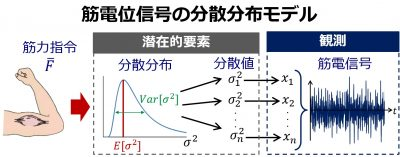# Resarch topics## Variance Distribution Model of Surface EMG Signals Based on Inverse Gamma DistributionBioelectric signal group

This study formulated a surface EMG model capable of representing the variance distribution of EMG signals. In the model, EMG signals are handled based on a Gaussian white noise process with a mean of zero for each variance value. EMG signal variance is taken as a random variable that follows an inverse gamma distribution, allowing the representation of noise superimposed onto this variance. The estimation of the variance distribution based on marginal likelihood maximization was also proposed. The procedure can be approximated using rectified and smoothed EMG signals, thereby allowing the determination of distribution parameters in real time at low computational cost. The variance distribution estimated using the proposed model exhibits potential for the estimation of muscle force.

### References

1. A Variance Distribution Model of Surface EMG Signals Based on Inverse Gamma Distribution, Hideaki Hayashi, Akira Furui, Yuichi Kurita, and Toshio Tsuji, IEEE Transactions on Biomedical Engineering (Forthcoming 2017)

2. Variance Distribution Analysis of Surface EMG Signals Based on Marginal Maximum Likelihood Estimation, Akira Furui, Hideaki Hayashi, Yuichi Kurita, and Toshio Tsuji, 39th Annual International Conference of the IEEE Engineering in Medicine and Biology Society (EMBC’17), Jeju Island, Korea, July 11-15, 2017